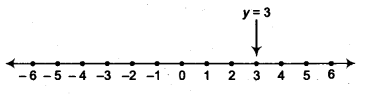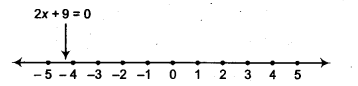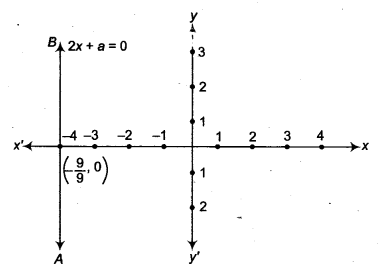NCERT Solutions for Class 9 Maths Chapter 8 Linear Equations in Two Variables Ex 8.4 are part of NCERT Solutions for Class 9 Maths. Here we have given NCERT Solutions for Class 9 Maths Chapter 8 Linear Equations in Two Variables Ex 8.4.

 Board CBSE Textbook NCERT Class Class 9 Subject Maths Chapter Chapter 8 Chapter Name Linear Equations in Two Variables Exercise Ex 8.4 Number of Questions Solved 2 Category NCERT Solutions

## NCERT Solutions for Class 9 Maths Chapter 8 Linear Equations in Two Variables Ex 8.4

Question 1.
Give the geometric representations of y = 3 as an equation
(i) in one variable.
(ii) in two variables.
Solution:
The given linear equation is
y=3 …(i)
(i) The representation of the solution on the number line is shown in the figure below, where y = 3 is treated as an equation in one variable.(ii) We know that, y = 3 can be written as
0 . x + y = 3
which is a linear equation in the variables x and y. This is represented by a line. Now, all the values of x are permissible because 0 . x is always 0.
However, y must satisfy the equation y = 3.
Note that, the graph AB is a line parallel to the x-axis and at a distance of 3 units to the upper side of it.Question 2.
Give the geometric representations of 2x + 9 = 0 as an equation
(i) in one variable.
(ii) in two variables.
Solution:
The given linear equation is
2x + 9=0
⇒ x = –$$\frac { 9 }{ 2 }$$ …. ( i)
(i) The representation of the solution on the number line is shown in the figure below, where x = –$$\frac { 9 }{ 2 }$$ is treated as an equation in one variable.(ii) We know that, 2x + 9= 0 can be written as
2x + 0y + 9 = 0
which is a linear equation in two variables x and y.
This is represented by a line.
Now, all the values of y are permissible because 0 .
y is always 0.
However, x must satisfy the equation 2x + 9 = 0.
Note that, the graph AB is a line parallel to the y-axis and at a distance of – $$\frac { 9 }{ 2 }$$ = – 4.5 to the left of it.We hope the NCERT Solutions for Class 9 Maths Chapter 8 Linear Equations in Two Variables Ex 8.4 help you. If you have any query regarding NCERT Solutions for Class 9 Maths Chapter 8 Linear Equations in Two Variables Ex 8.4, drop a comment below and we will get back to you at the earliest.